# [Python知识图谱] 四.Python和Gephi实现中国知网合作关系知识图谱

1.在中国知网搜索“清水江”关键词，并导出论文Excel格式。
2.使用Python处理文本，获取作者合作的共现矩阵及三元组。
3.Gephi导入CSV节点及边文件，并构建关系图谱。
4.Gephi调整参数，优化关系图谱。

# 一.最终效果图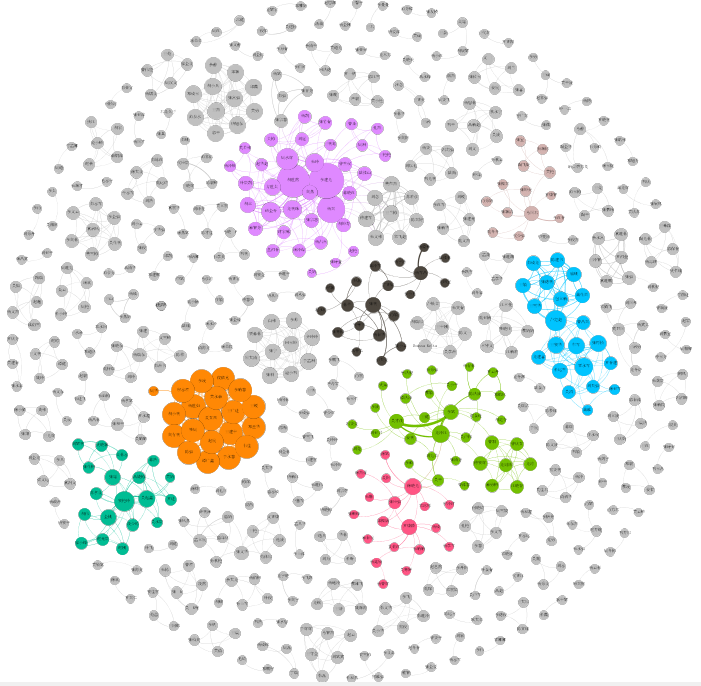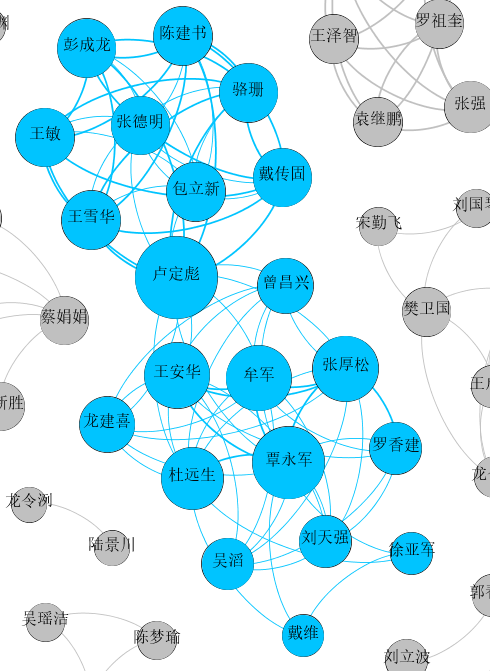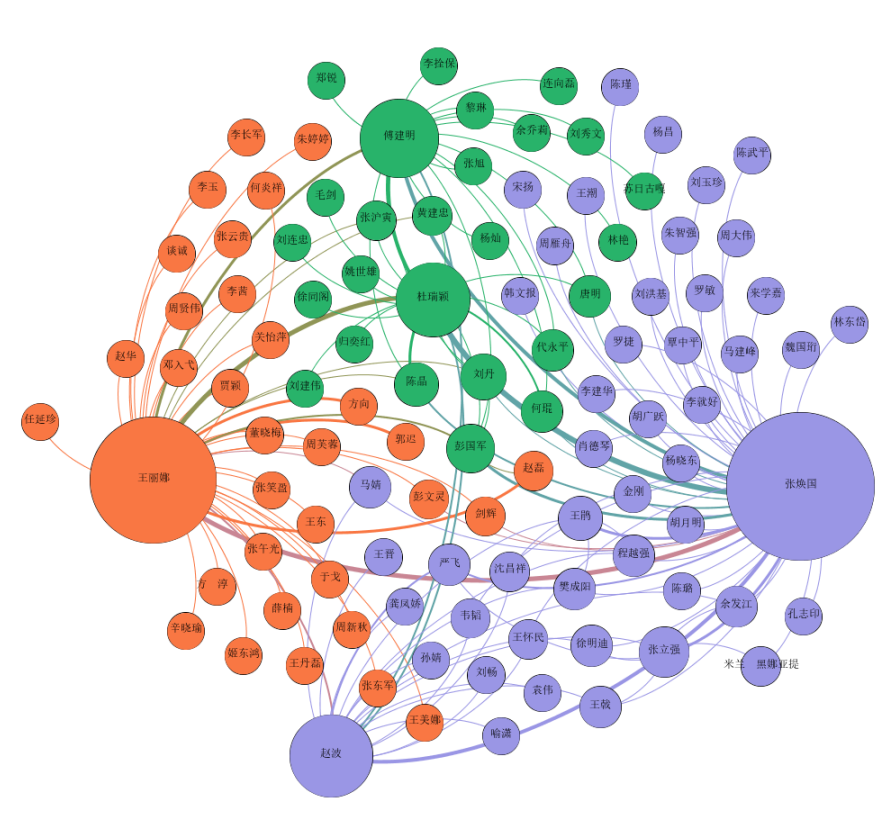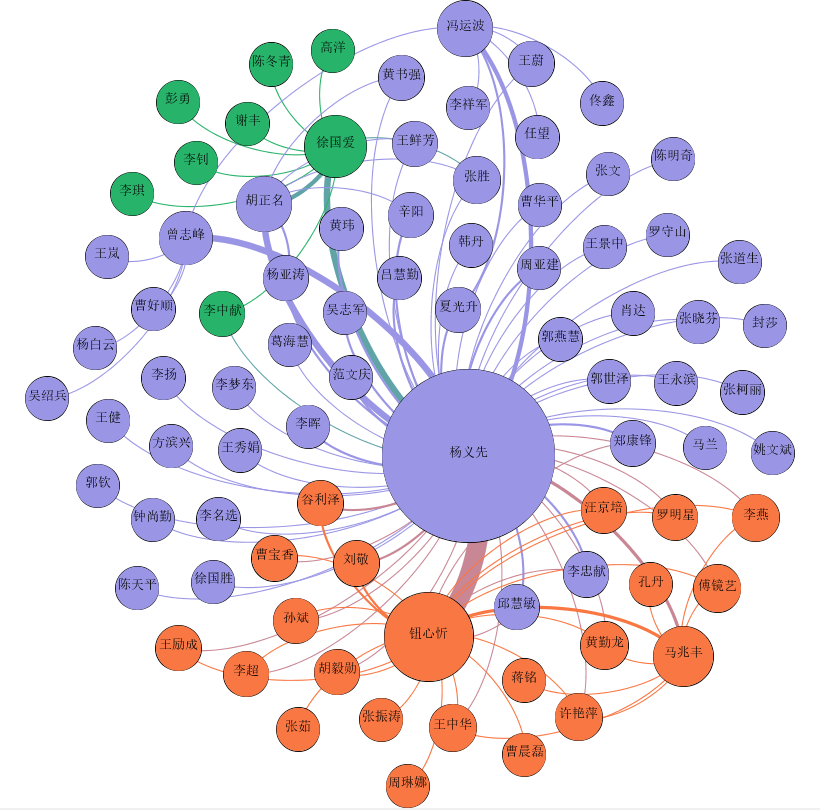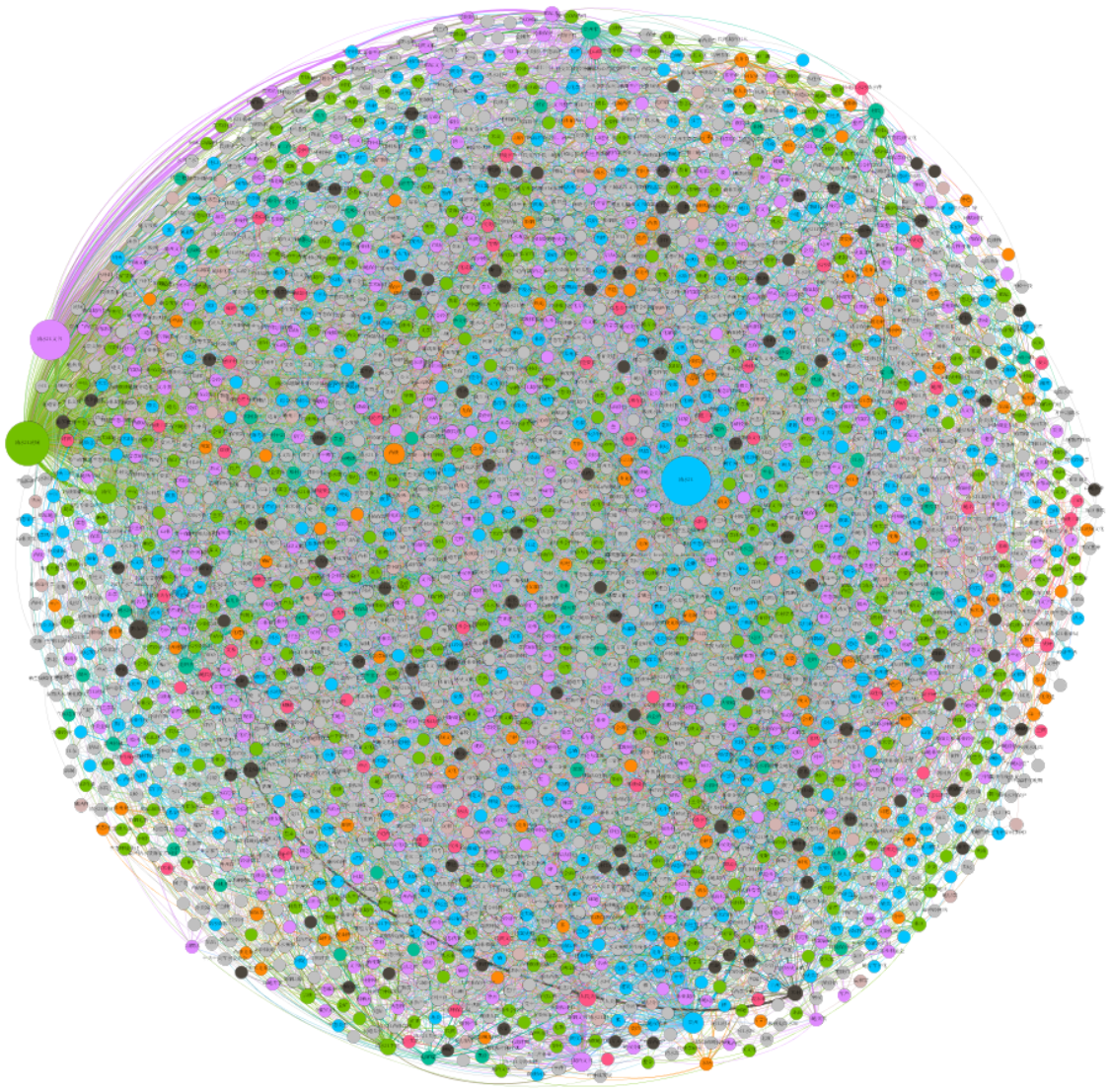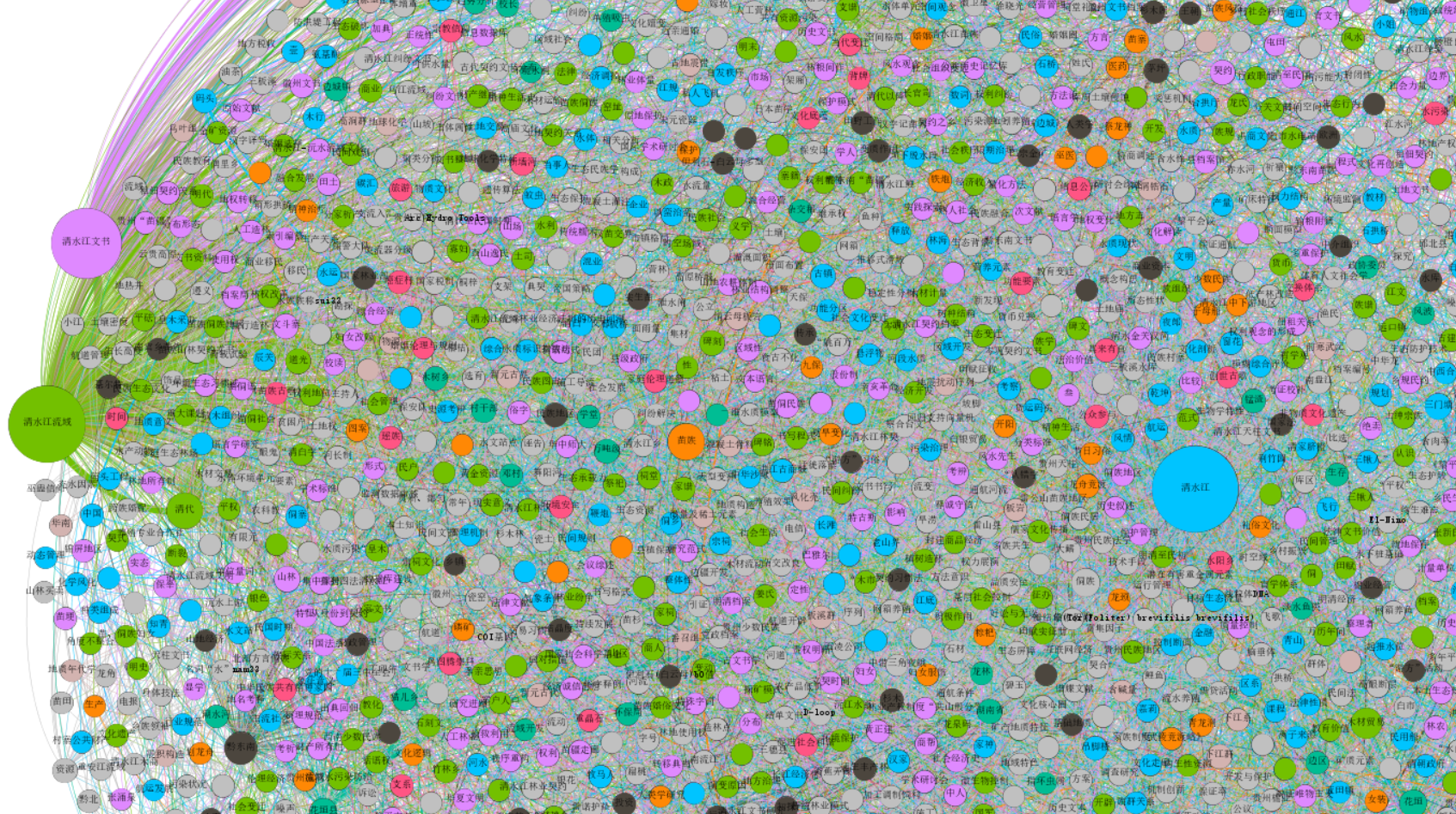# 二.中国知网导出数据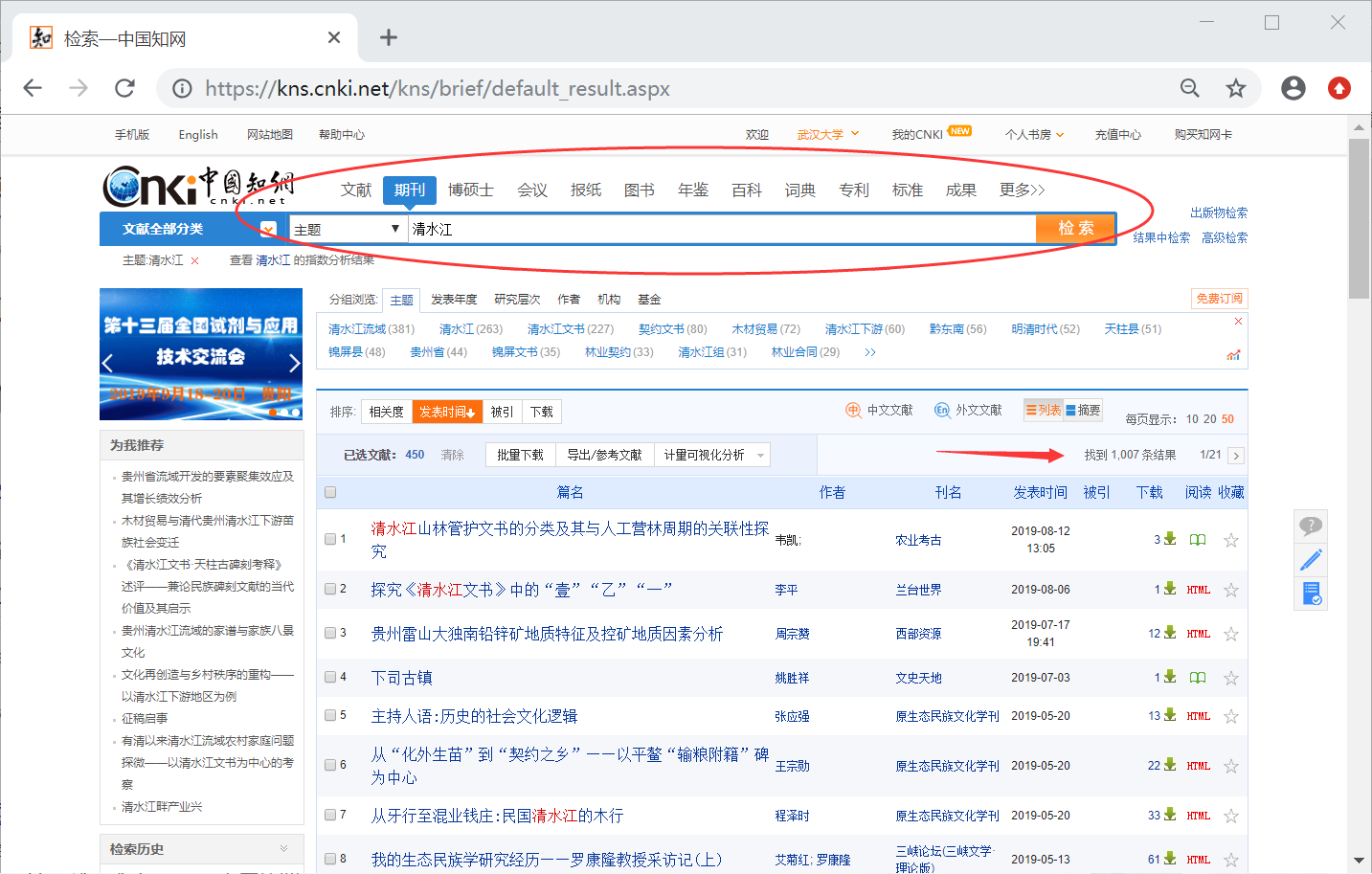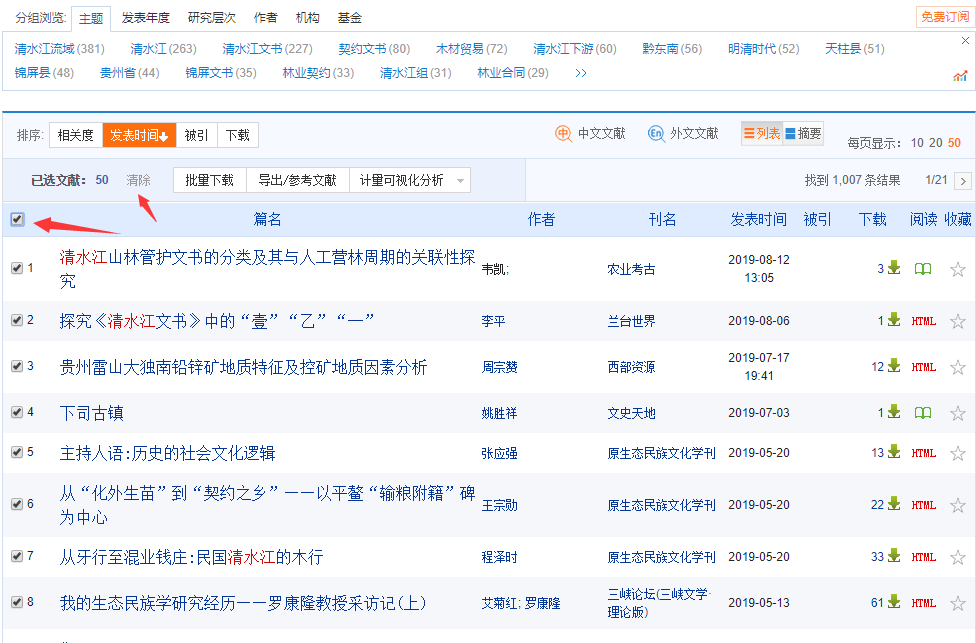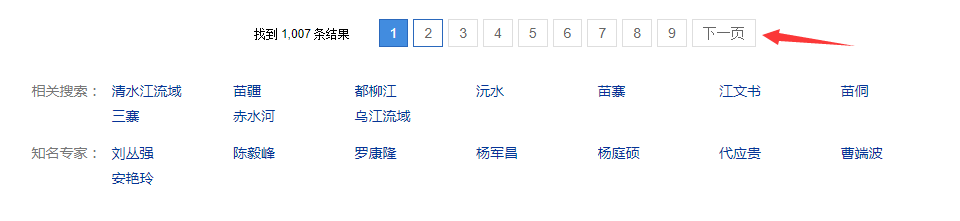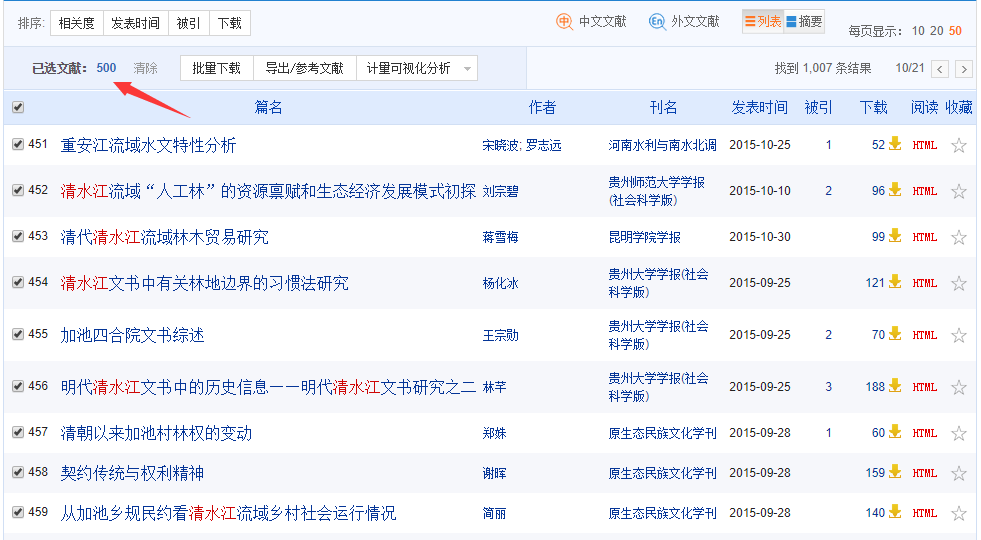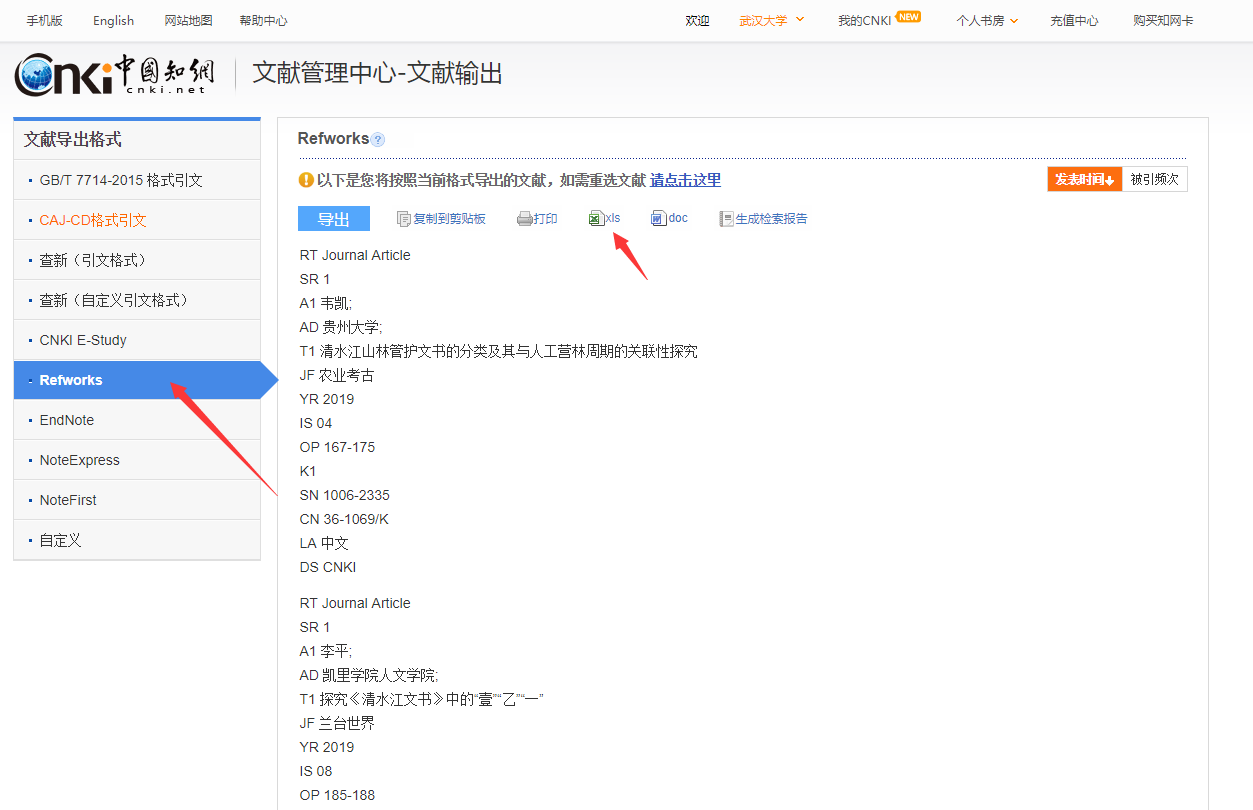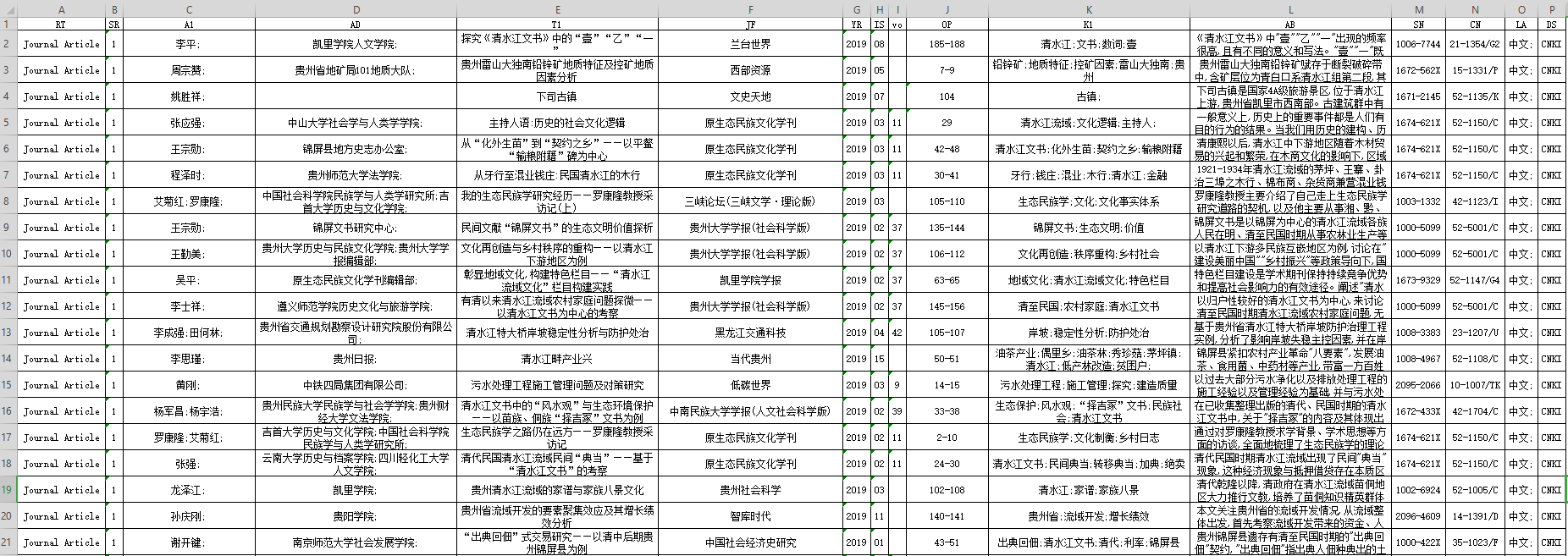# 三.什么是共现关系

文章标题                                        作者

Python网络爬虫                                 A，B，C



$\left[ \begin{matrix} -& A & B & C & D \\ A & 0 & 2 & 2 & 2 \\ B & 1 & 0 & 2 & 2 \\ C & 0 & 0 & 0 & 1 \\ D & 1 & 0 & 0 & 0 \end{matrix} \right] \tag{1}$

$\left[ \begin{matrix} -& A & B & C & D \\ A & 0 & 3 & 2 & 3 \\ B & 0 & 0 & 2 & 2 \\ C & 0 & 0 & 0 & 1 \\ D & 0 & 0 & 0 & 0 \end{matrix} \right] \tag{1}$

$\left[ \begin{matrix} Source & Target & Weight \\ A & B & 3 \\ A & C & 2 \\ A & D & 3 \\ B & C & 2 \\ B & D & 2 \\ C & D & 1 \end{matrix} \right] \tag{2}$

# -*- coding: utf-8 -*-
import networkx as nx
import matplotlib.pyplot as plt

#定义有向图
DG = nx.Graph()
#添加五个节点(列表)
DG.add_nodes_from(['A', 'B', 'C', 'D'])
print DG.nodes()
#添加边(列表)
#DG.add_edges_from([('A', 'B'), ('A', 'C'), ('A', 'D'), ('B','C'),('B','D'),('C','D')])
print DG.edges()
#绘制图形 设置节点名显示\节点大小\节点颜色
colors = ['red', 'green', 'blue', 'yellow']
nx.draw(DG,with_labels=True, node_size=900, node_color = colors)
plt.show()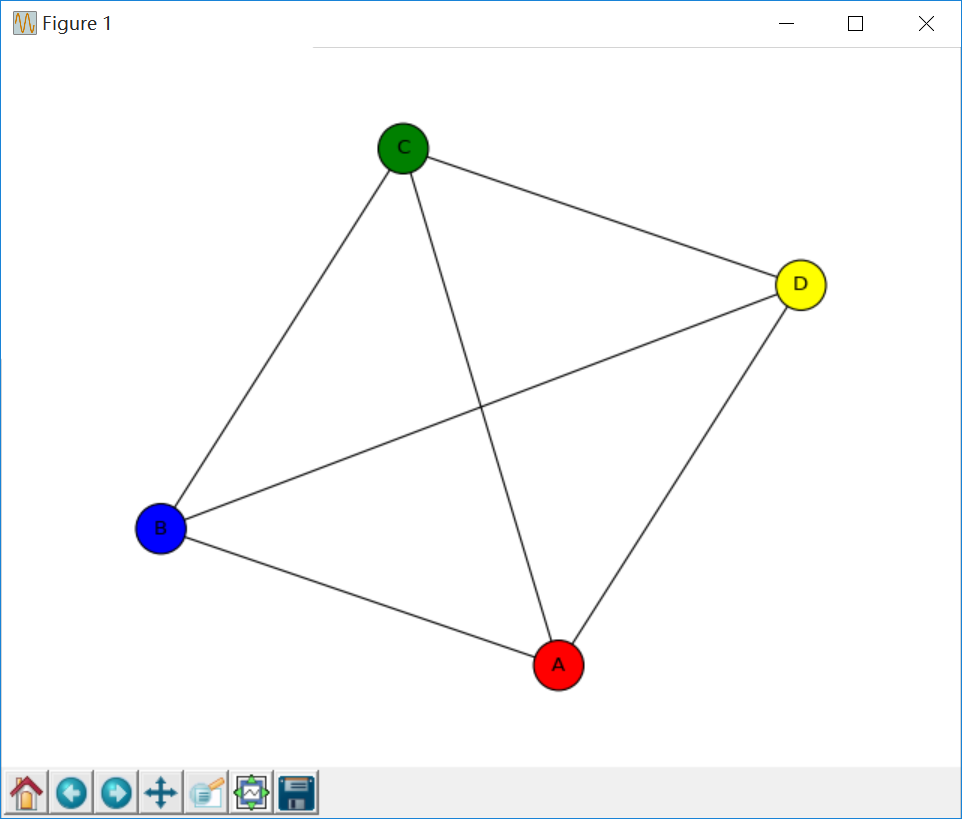# 四.Python构建共现矩阵和三元组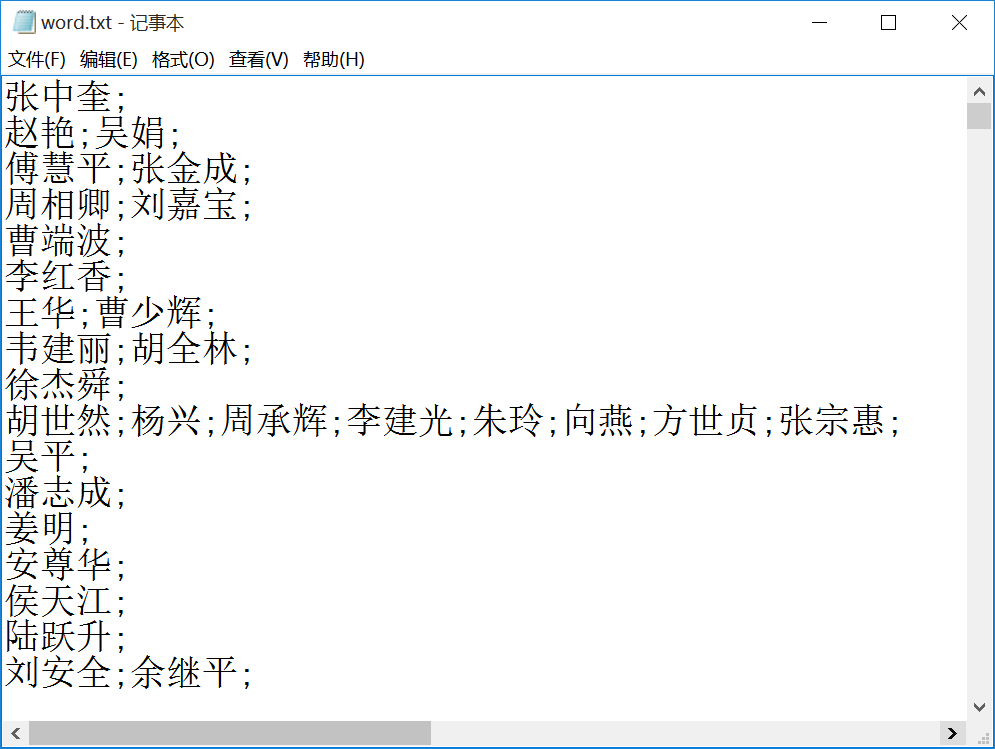• 按行读取TXT数据，并调用split(’;’)分割作者，将所有作者信息存储至word列表中（不含重复项）
• 建立 word_vector[作者数][作者数] 共现矩阵，依次获取每行的两个作者下标位置，记录在变量w1、w2、n1、n2中
• 词频矩阵赋值，这里通过判断计算上三角矩阵 word_vector[n1][n2] += 1 或 word_vector[n2][n1] += 1
• 两层循环获取共现矩阵 word_vector[i][j] 中不为0的数值，word[i]、word[j] 和权重 word_vector[i][j] 即为三元组
• 将最终结果输出至TXT和CSV文件
# -*- coding: utf-8 -*-
"""
@author: eastmount CSDN 杨秀璋 2019-09-02
"""
import pandas as pd
import numpy as np
import codecs
import networkx as nx
import matplotlib.pyplot as plt
import csv

#---------------------------第一步:读取数据-------------------------------
word = [] #记录关键词
f = open("word.txt")
while line:
#print line
line = line.replace("\n", "") #过滤换行
line = line.strip('\n')
for n in line.split(';'):
#print n
if n not in word:
word.append(n)
f.close()
print len(word) #作者总数

#--------------------------第二步 计算共现矩阵----------------------------
a = np.zeros([2,3])
print a

#共现矩阵
#word_vector = np.zeros([len(word),len(word)], dtype='float16')

#MemoryError：矩阵过大汇报内存错误
#https://jingyan.baidu.com/article/a65957f434970a24e67f9be6.html
#采用coo_matrix函数解决该问题

from scipy.sparse import coo_matrix
print len(word)
#类型<type 'numpy.ndarray'>
word_vector = coo_matrix((len(word),len(word)), dtype=np.int8).toarray()
print word_vector.shape

f = open("word.txt")
while line:
line = line.replace("\n", "") #过滤换行
line = line.strip('\n') #过滤换行
nums = line.split(';')

#循环遍历关键词所在位置 设置word_vector计数
i = 0
j = 0
while i<len(nums):       #ABCD共现 AB AC AD BC BD CD加1
j = i + 1
w1 = nums[i]           #第一个单词
while j<len(nums):
w2 = nums[j]       #第二个单词
#从word数组中找到单词对应的下标
k = 0
n1 = 0
while k<len(word):
if w1==word[k]:
n1 = k
break
k = k +1
#寻找第二个关键字位置
k = 0
n2 = 0
while k<len(word):
if w2==word[k]:
n2 = k
break
k = k +1
#重点: 词频矩阵赋值 只计算上三角
if n1<=n2:
word_vector[n1][n2] = word_vector[n1][n2] + 1
else:
word_vector[n2][n1] = word_vector[n2][n1] + 1
#print n1, n2, w1, w2
j = j + 1
i = i + 1
#读取新内容
f.close()

#--------------------------第三步  TXT文件写入--------------------------
res = open("word_word_weight.txt", "a+")
i = 0
while i<len(word):
w1 = word[i]
j = 0
while j<len(word):
w2 = word[j]
#判断两个词是否共现 共现&词频不为0的写入文件
if word_vector[i][j]>0:
#print w1 +" " + w2 + " "+ str(int(word_vector[i][j]))
res.write(w1 +" " + w2 + " "+ str(int(word_vector[i][j]))  +  "\r\n")
j = j + 1
i = i + 1
res.close()

#共现矩阵写入文件 如果作者数量较多, 建议删除下面部分代码
res = open("word_jz.txt", "a+")
i = 0
while i<len(word):
j = 0
jz = ""
while j<len(word):
jz = jz + str(int(word_vector[i][j])) + " "
j = j + 1
res.write(jz + "\r\n")
i = i + 1
res.close()

#--------------------------第四步  CSV文件写入--------------------------
c = open("word-word-weight.csv","wb")    #创建文件
#c.write(codecs.BOM_UTF8)                   #防止乱码
writer = csv.writer(c)                                #写入对象
writer.writerow(['Word1', 'Word2', 'Weight'])

i = 0
while i<len(word):
w1 = word[i]
j = 0
while j<len(word):
w2 = word[j]
#判断两个词是否共现 共现词频不为0的写入文件
if word_vector[i][j]>0:
#写入文件
templist = []
templist.append(w1)
templist.append(w2)
templist.append(str(int(word_vector[i][j])))
#print templist
writer.writerow(templist)
j = j + 1
i = i + 1
c.close()


A;B;C;D
A;B;C
B;A;D
D;A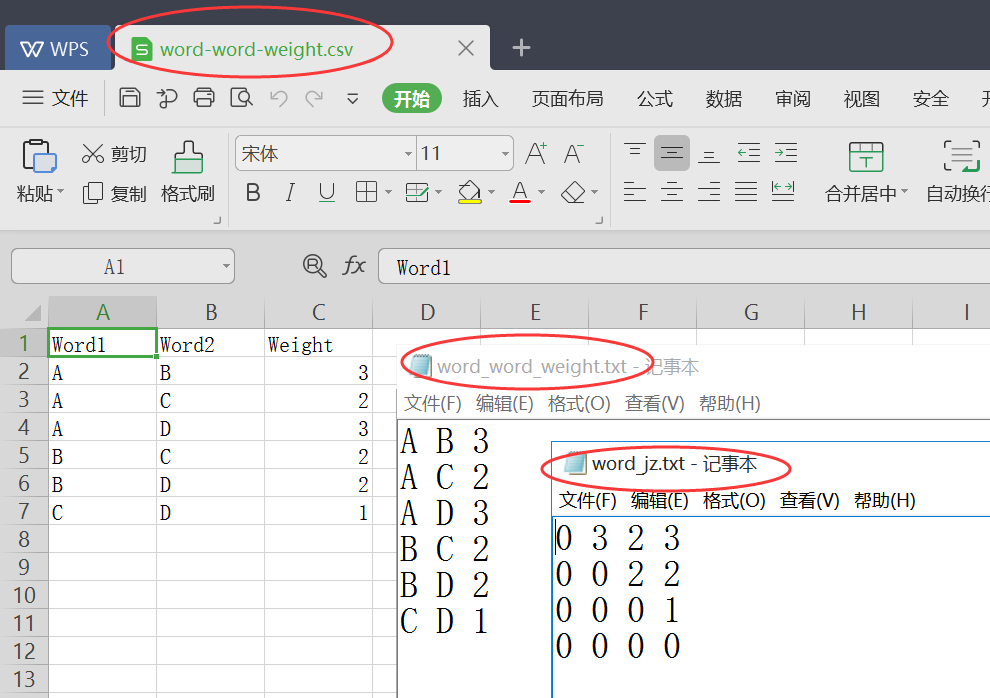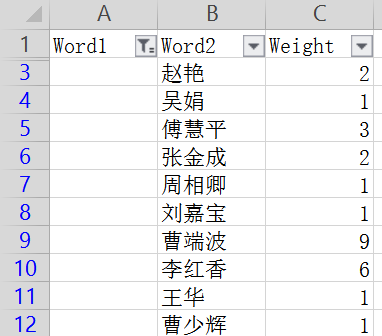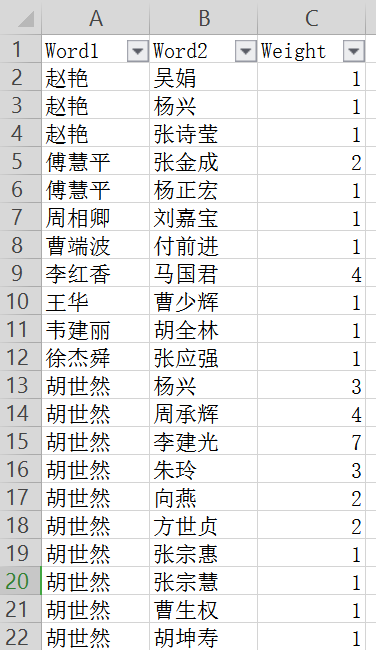# 五.Gephi构建知识图谱

## (一).数据准备

edges.csv

Source,Target,Type,Weight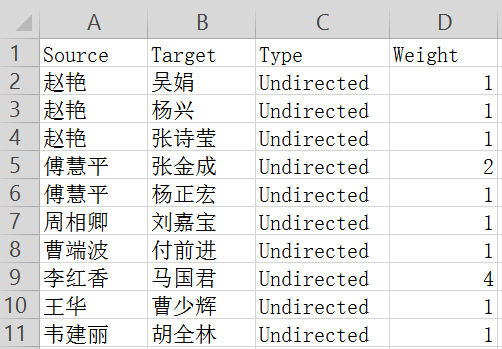nodes.csv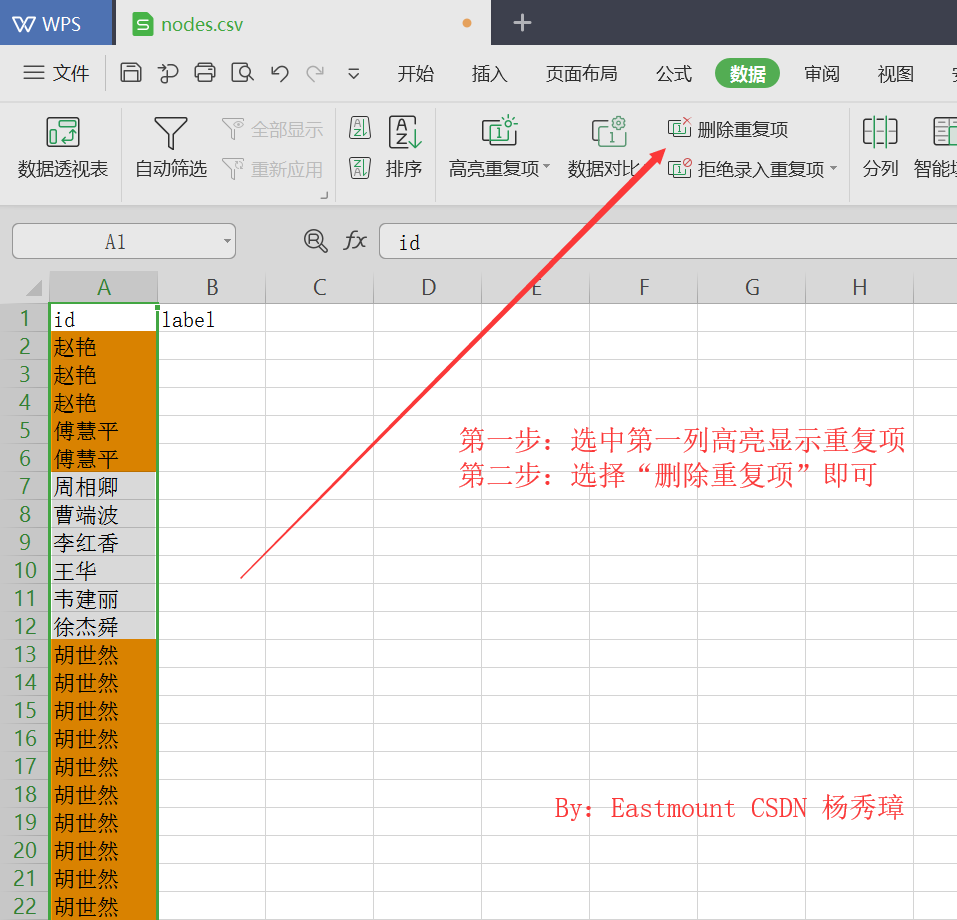id,label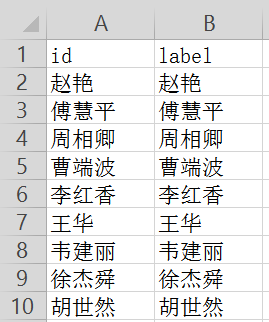## (二).数据导入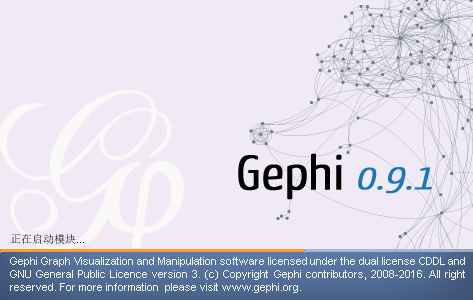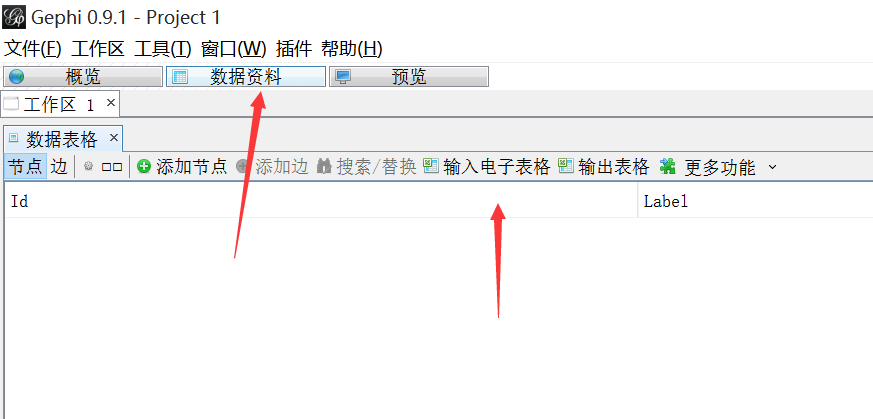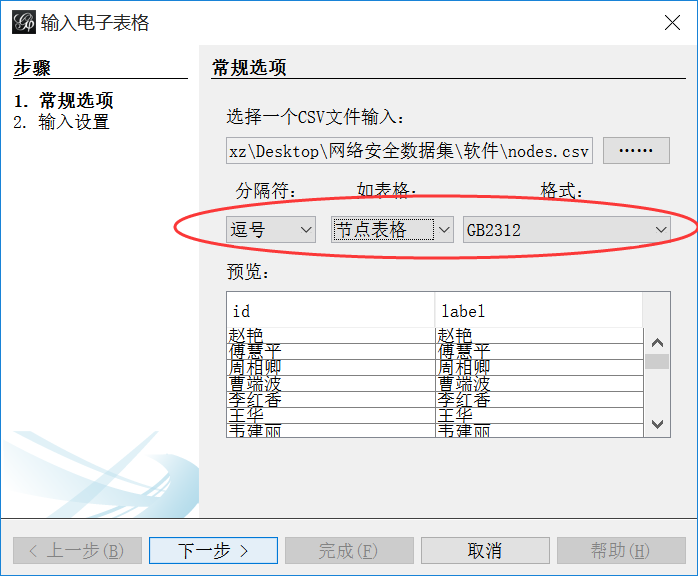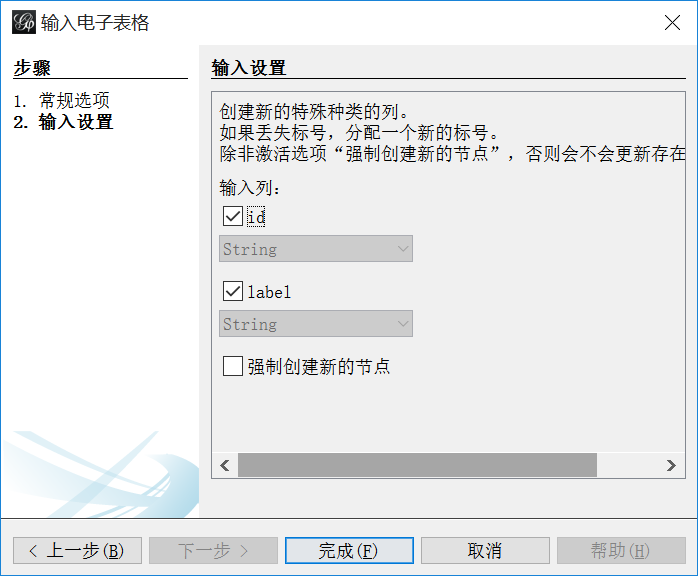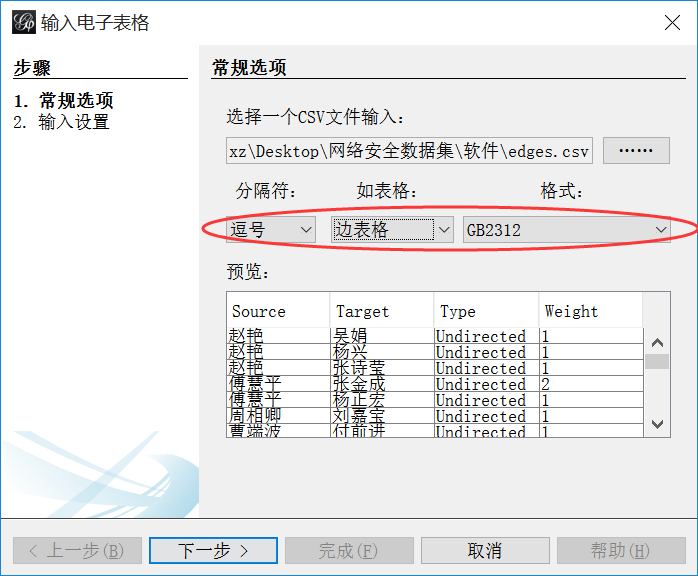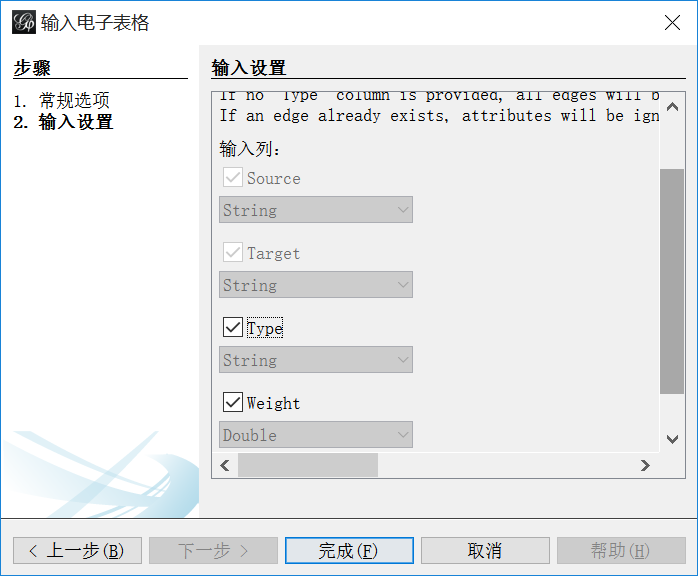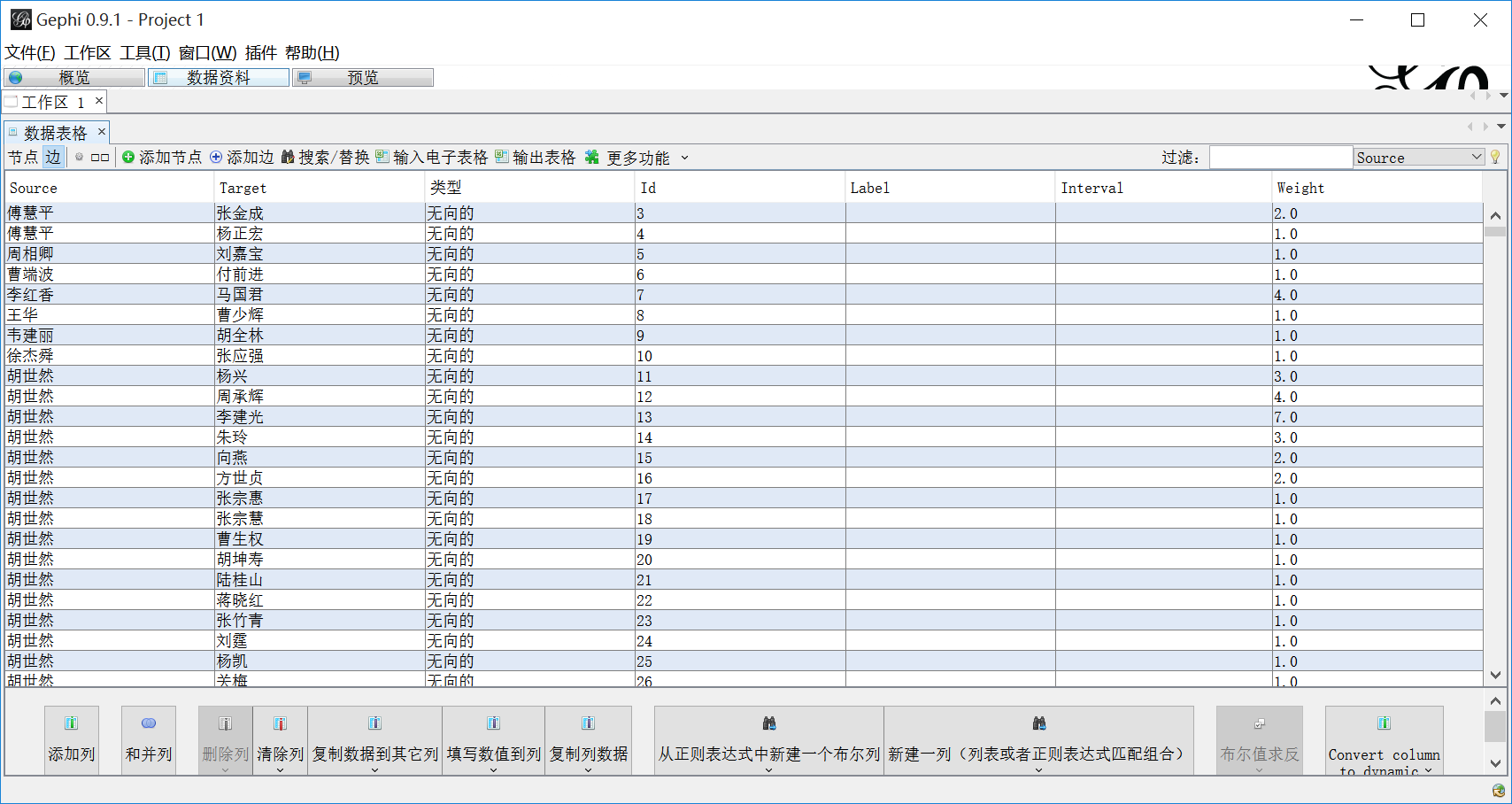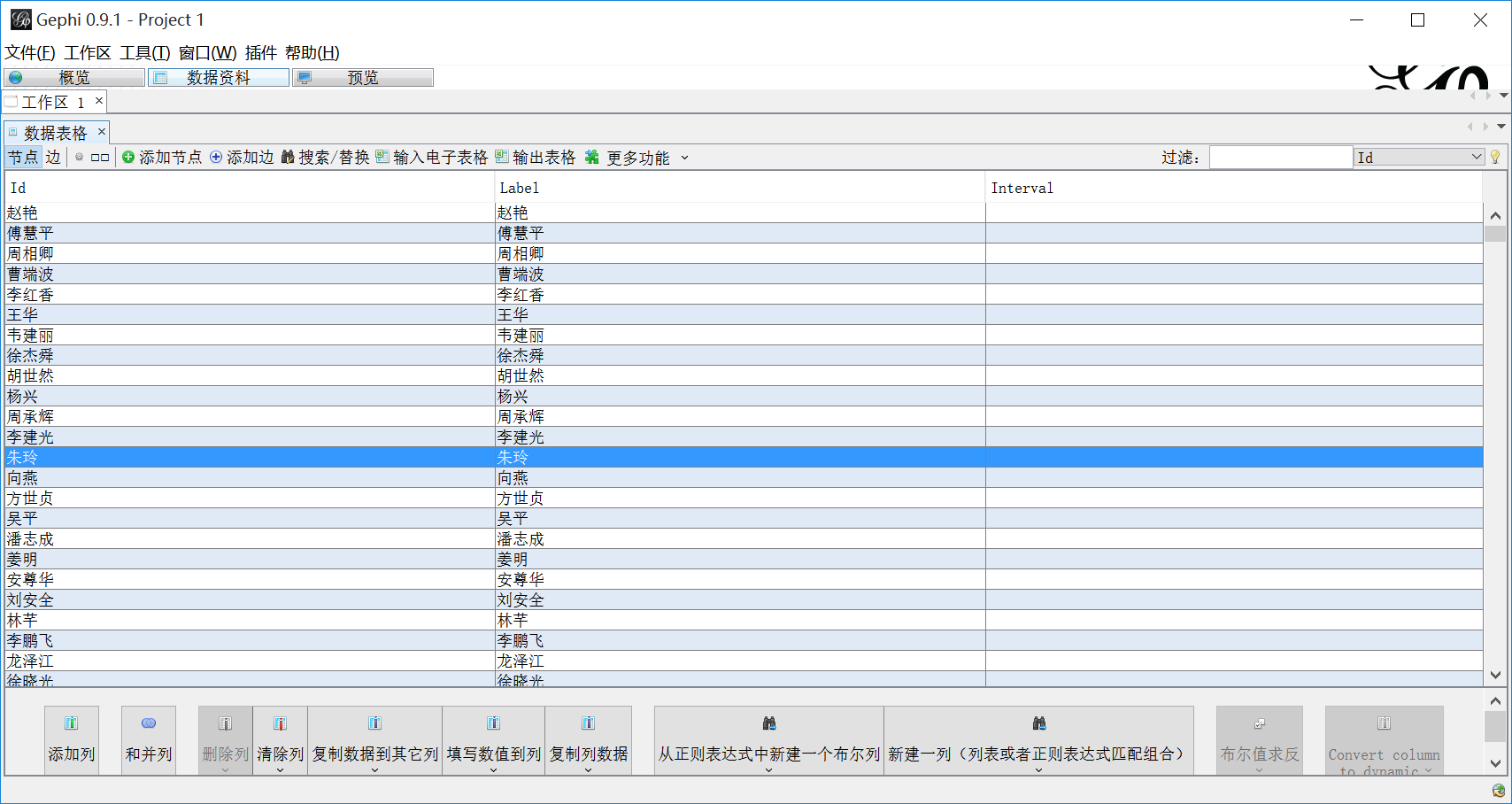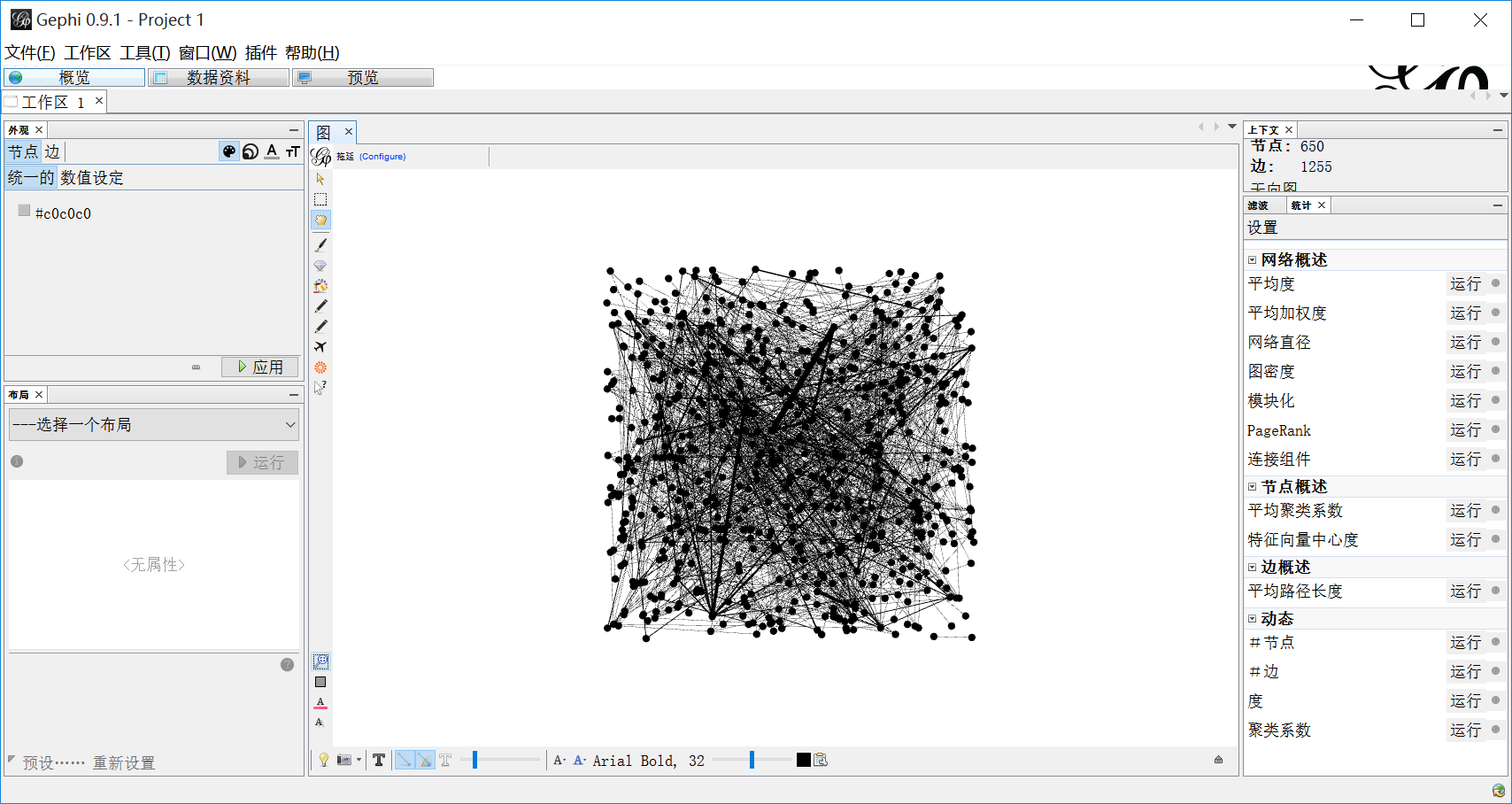## (三).知识图谱参数设置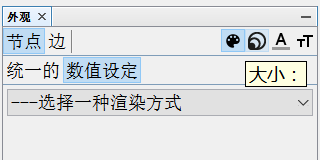1.设置节点大小和颜色。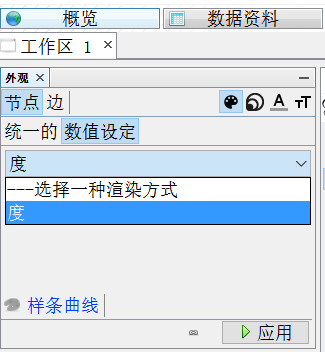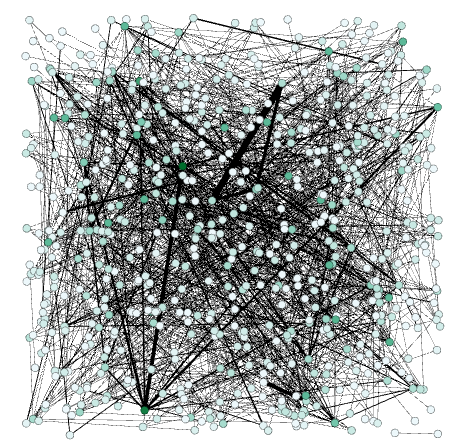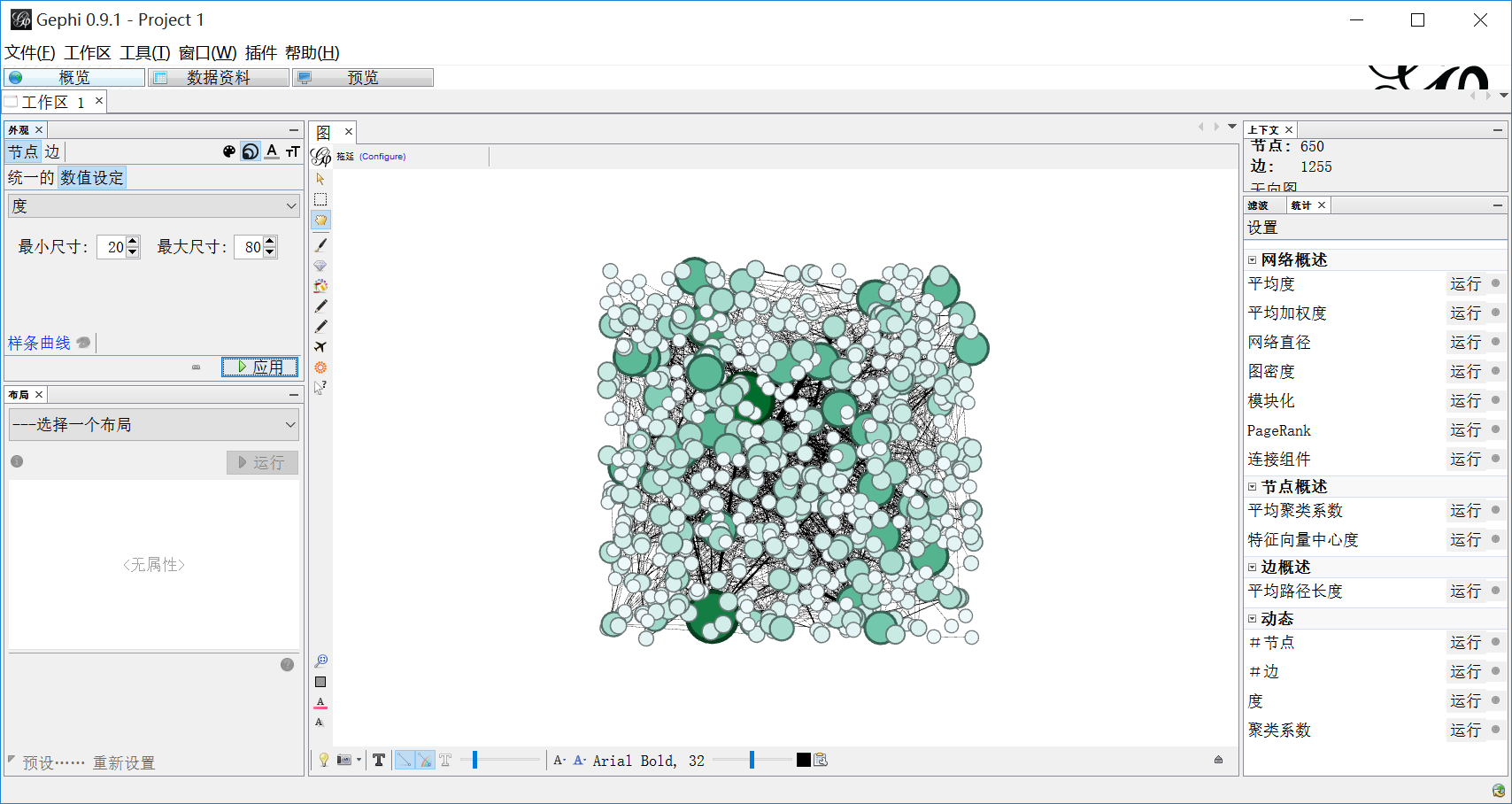2.设置边大小和颜色。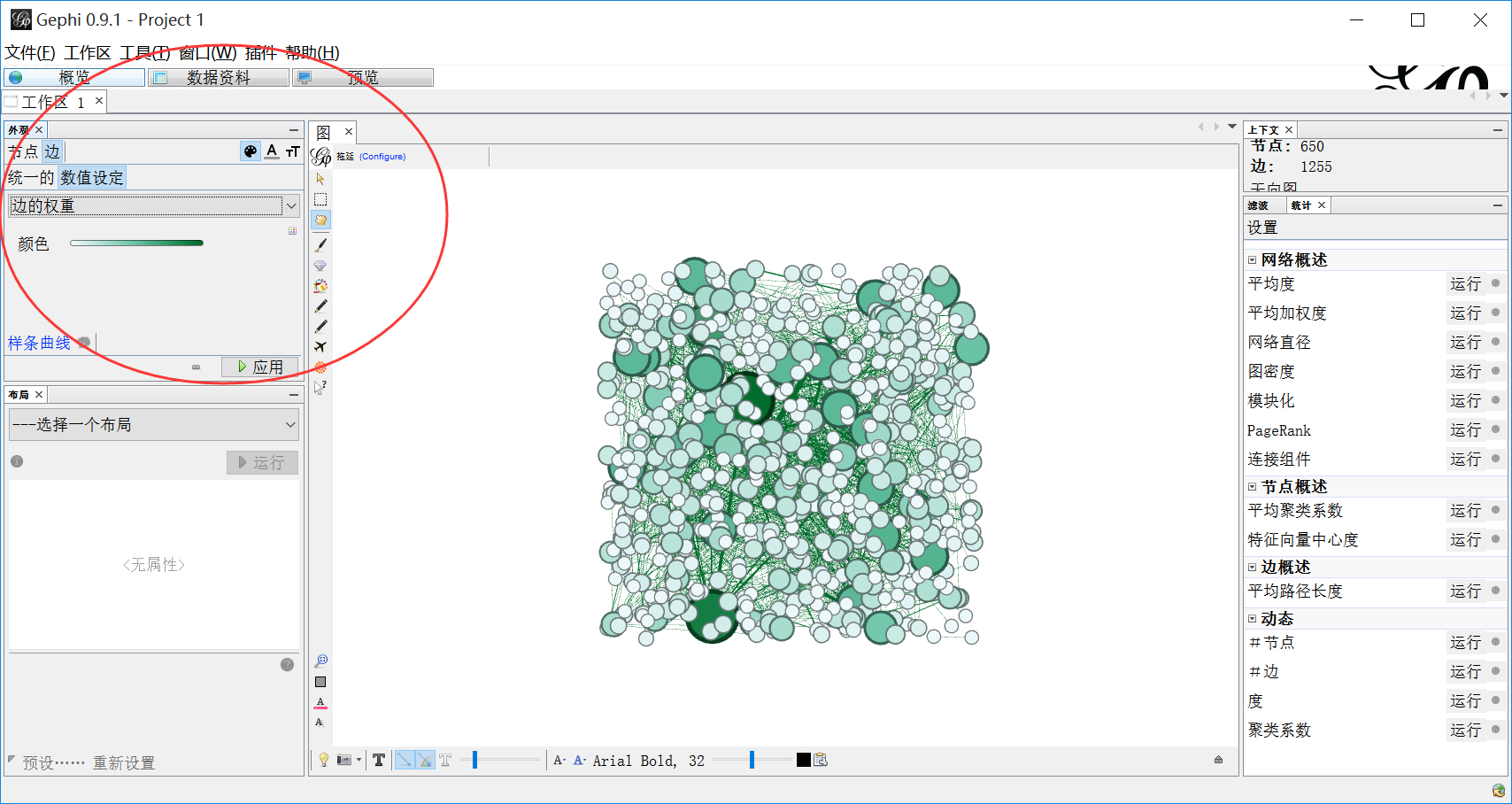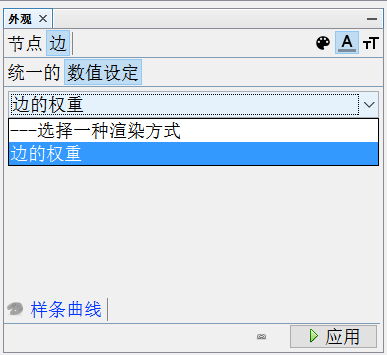3.在布局中选择“Fruchterman Reingold”。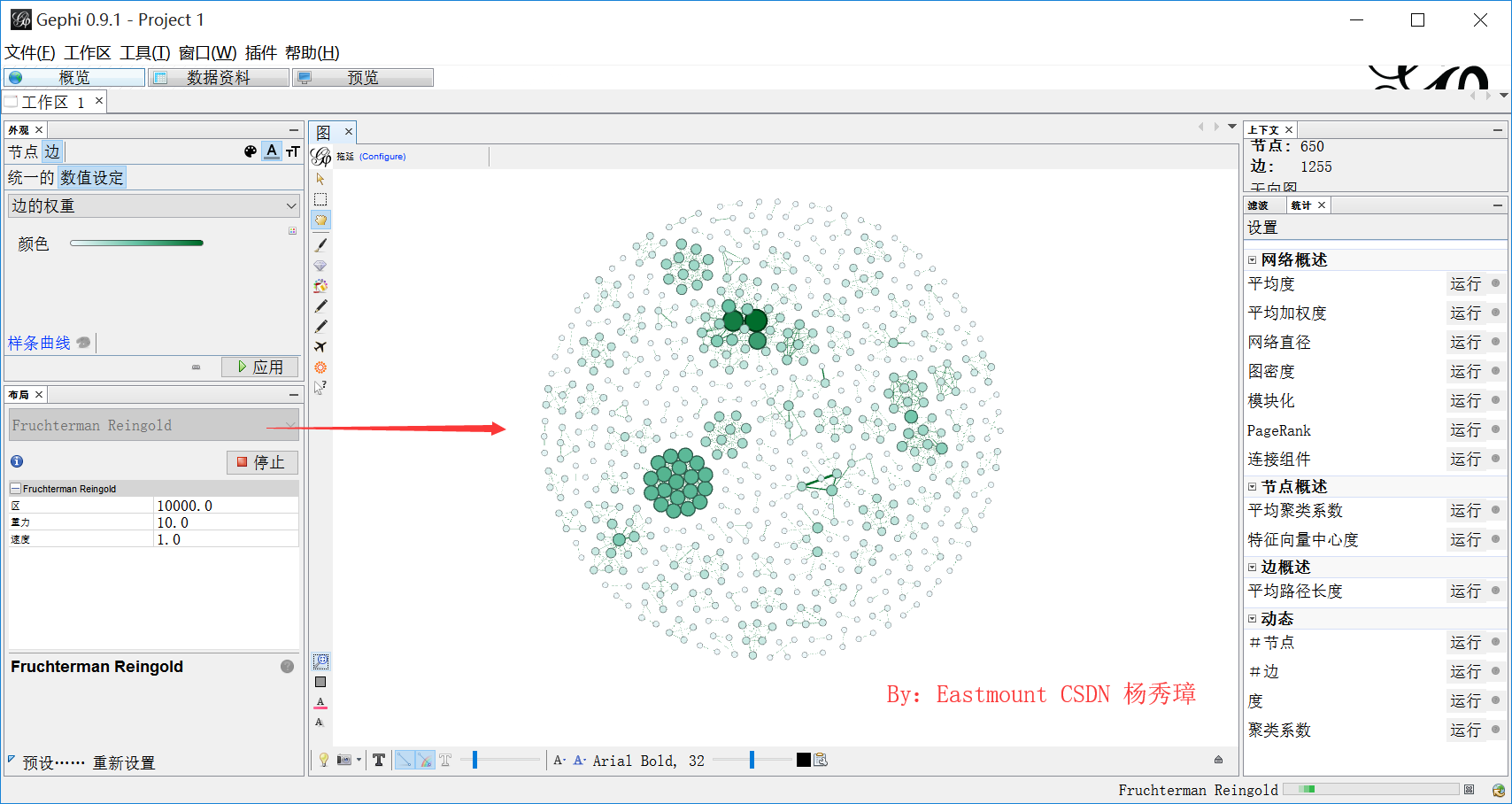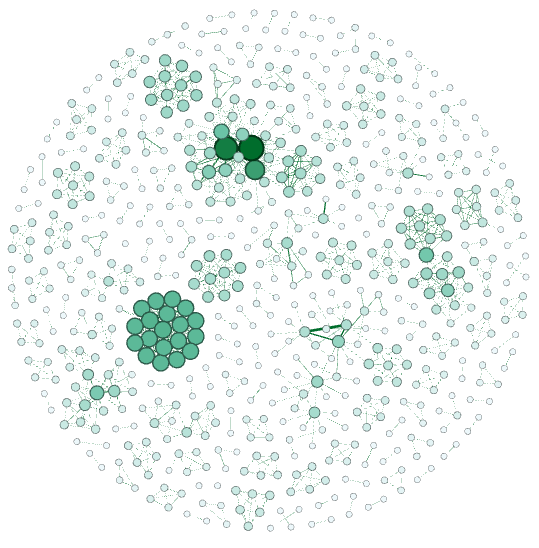4.设置模块化。在右边统计中点击“运行”，设置模块性。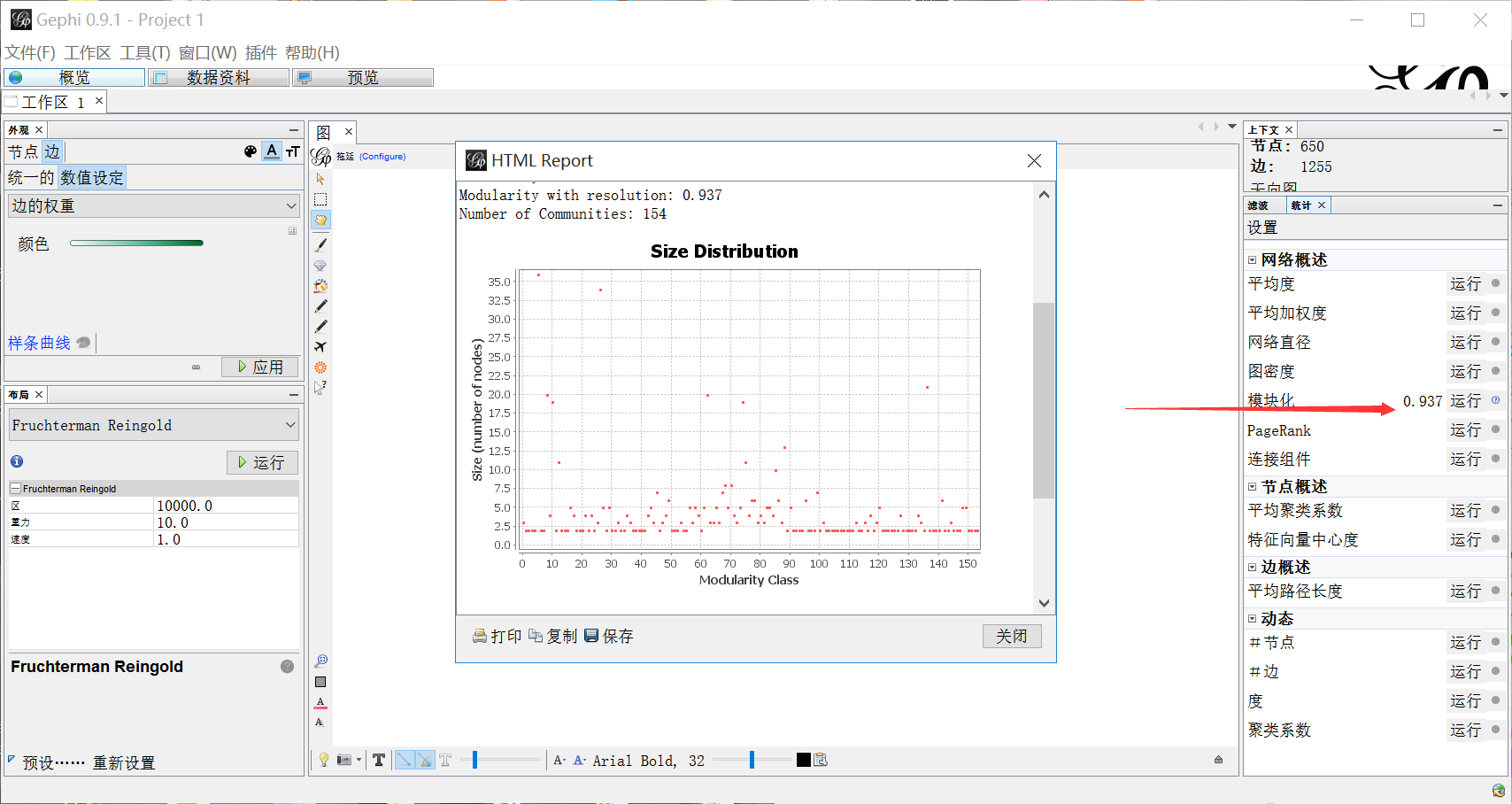5.设置平均路径长度。在右边统计中点击“运行”，设置边概述。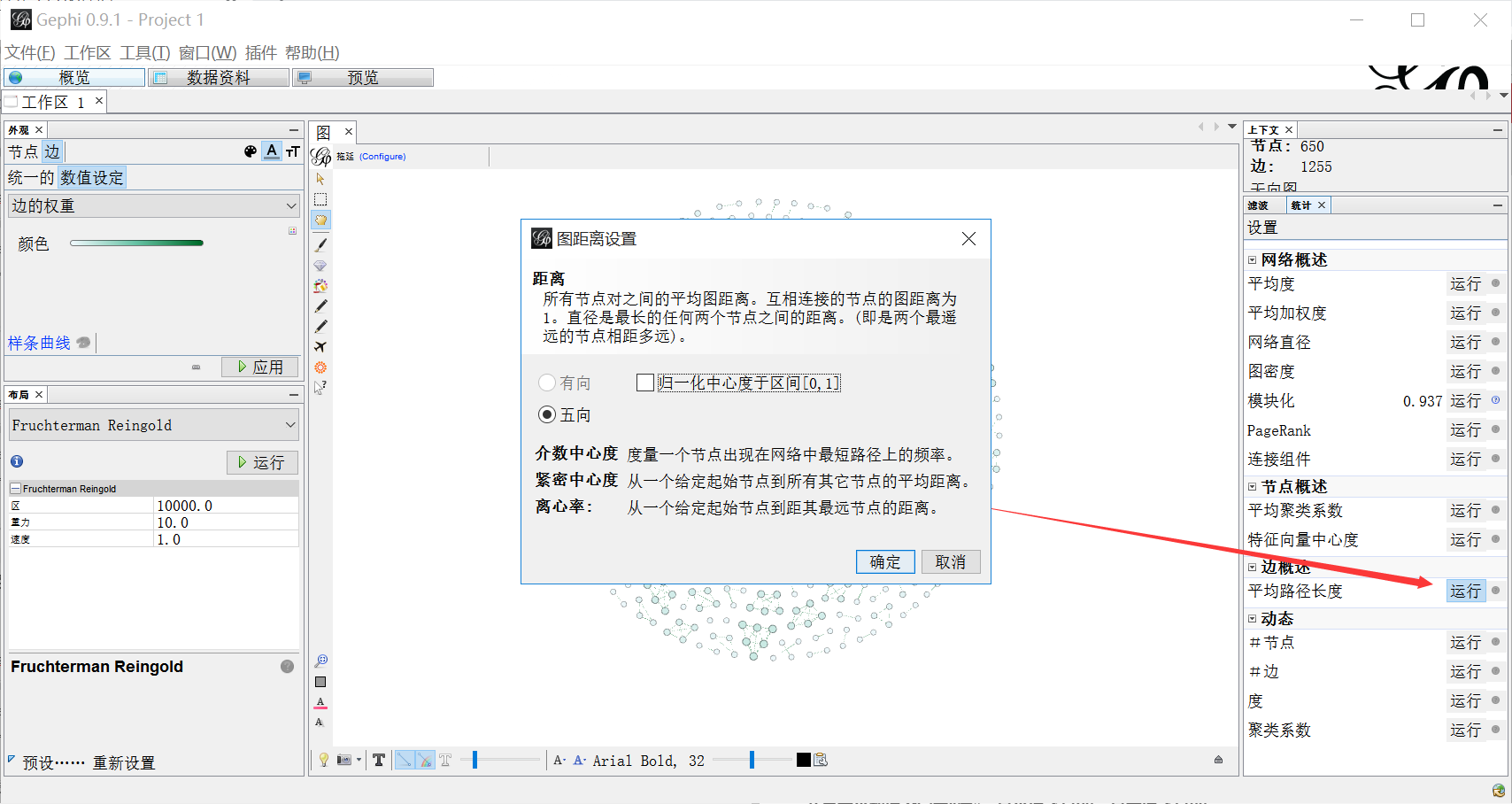6.重新设置节点属性。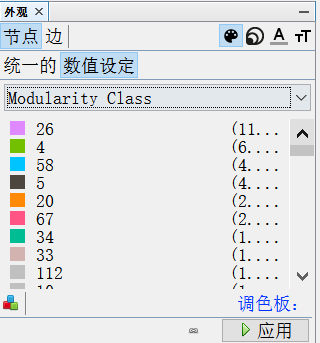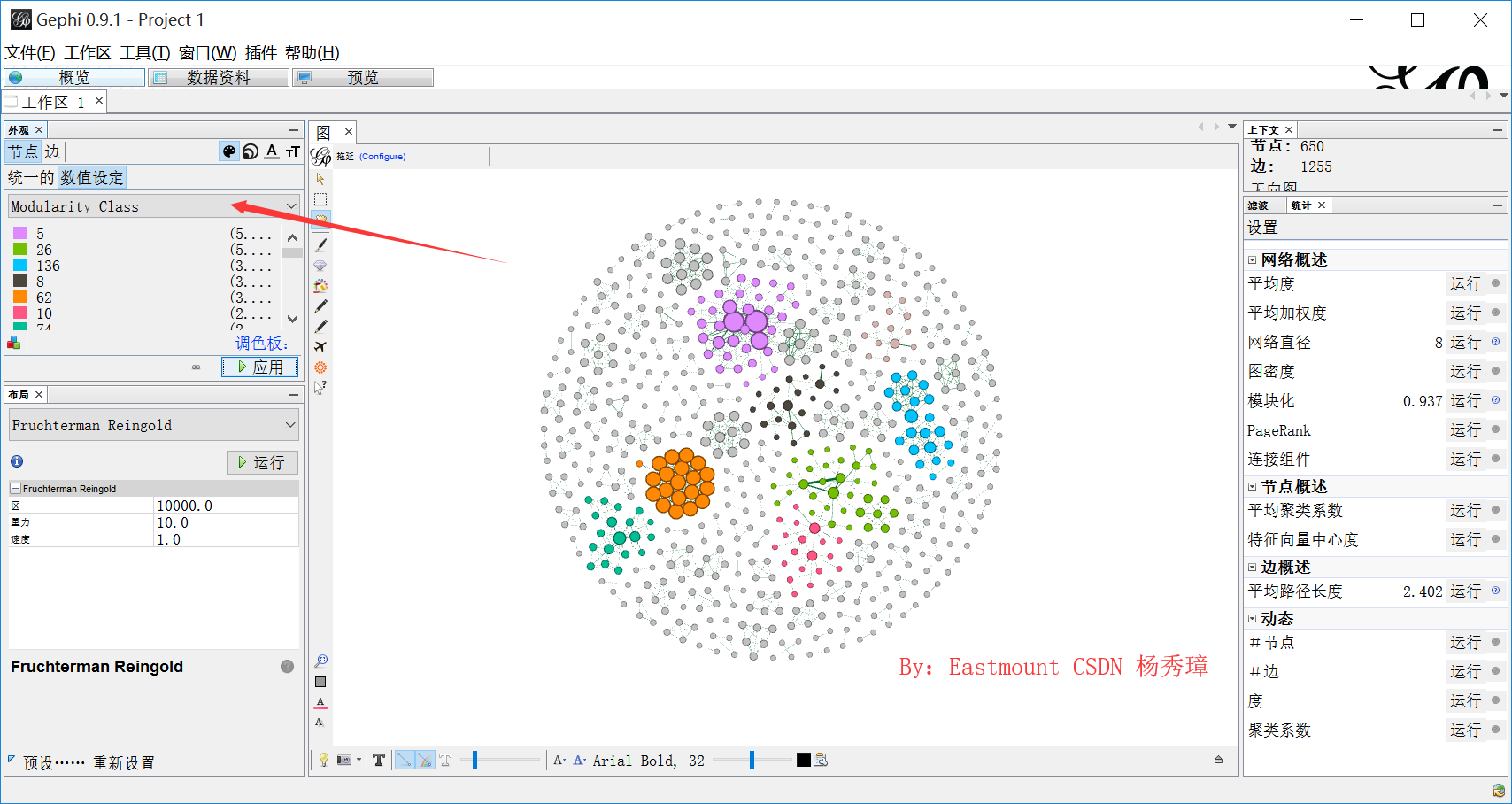## (四).可视化显示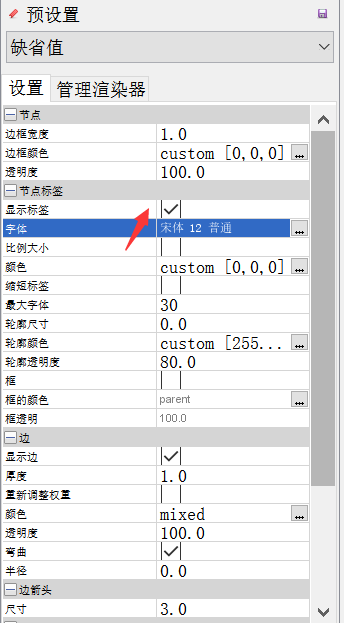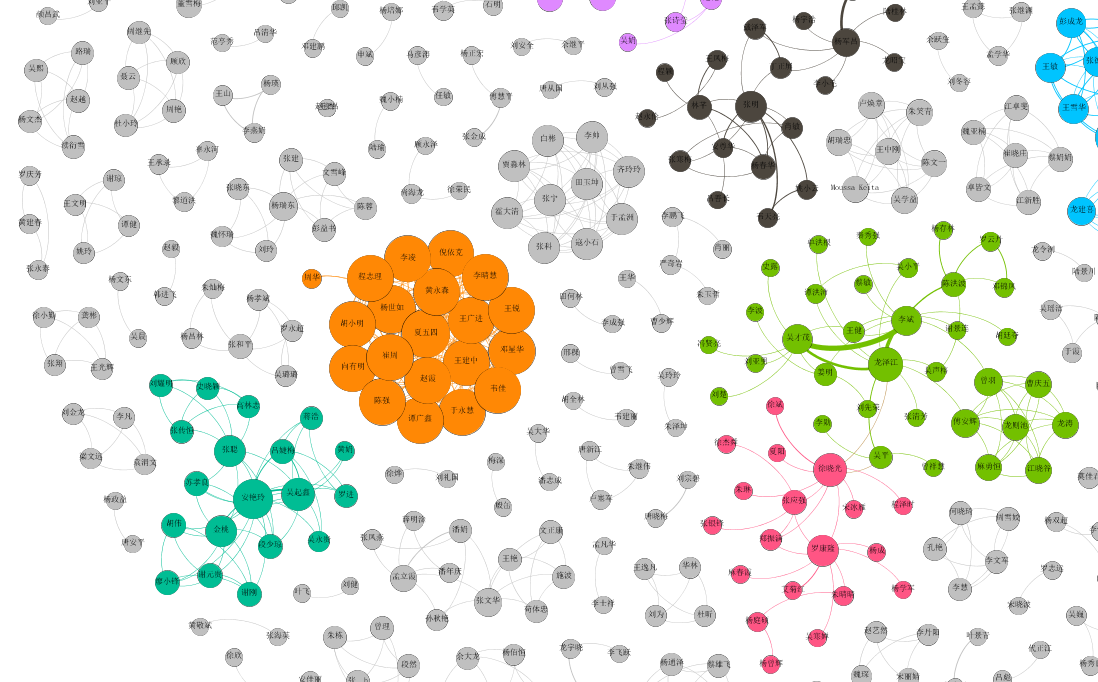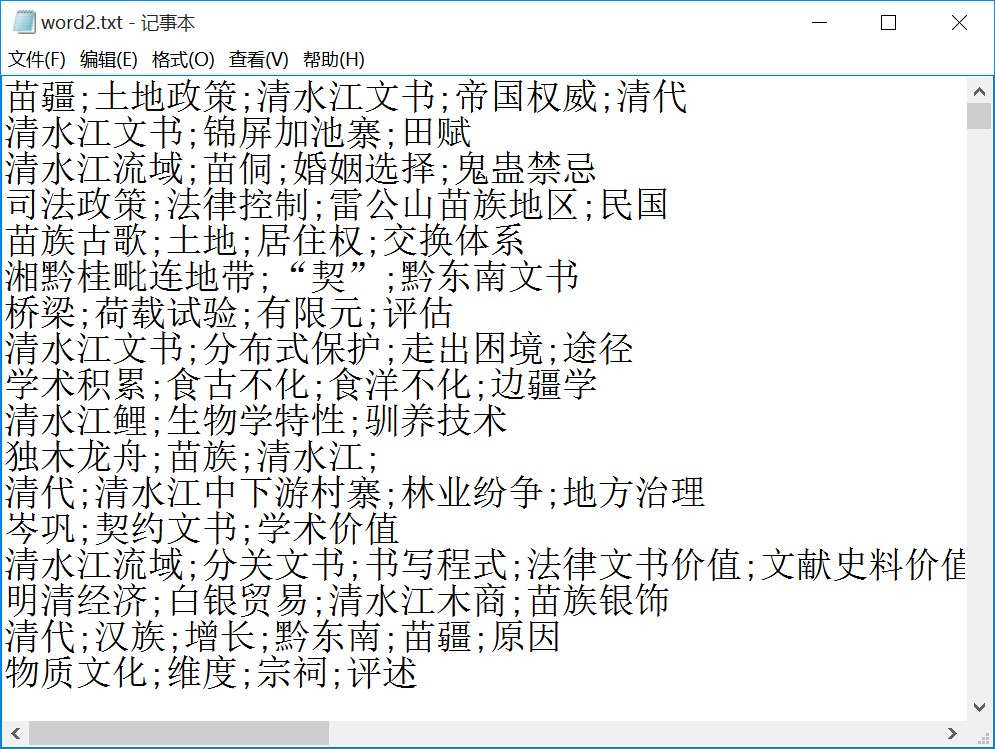# 六.总结(By:Eastmount 2019-09-02 下午5点 http://blog.csdn.net/eastmount/ )